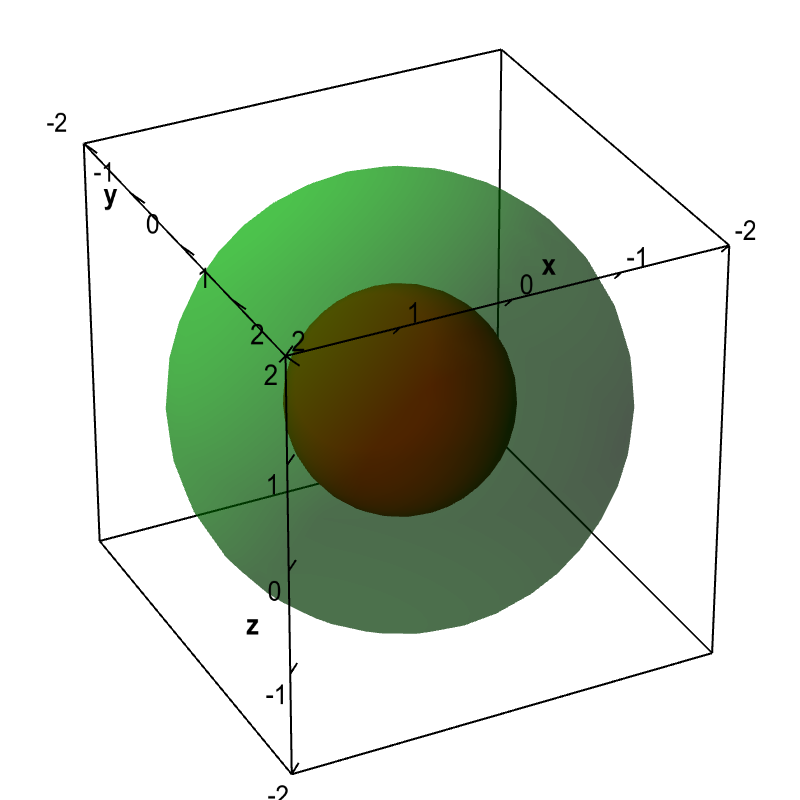# Math Insight

### Applet: Spherical level surfacesThe level surfaces $f(x,y,z) = x^2+y^2+z^2=c$ are spheres of radius $\sqrt{c}$. The level surface with $c=1$ is the sphere of radius 1 drawn in dark red. The level surface with $c=4$ is the sphere of radius 2 drawn in light green.